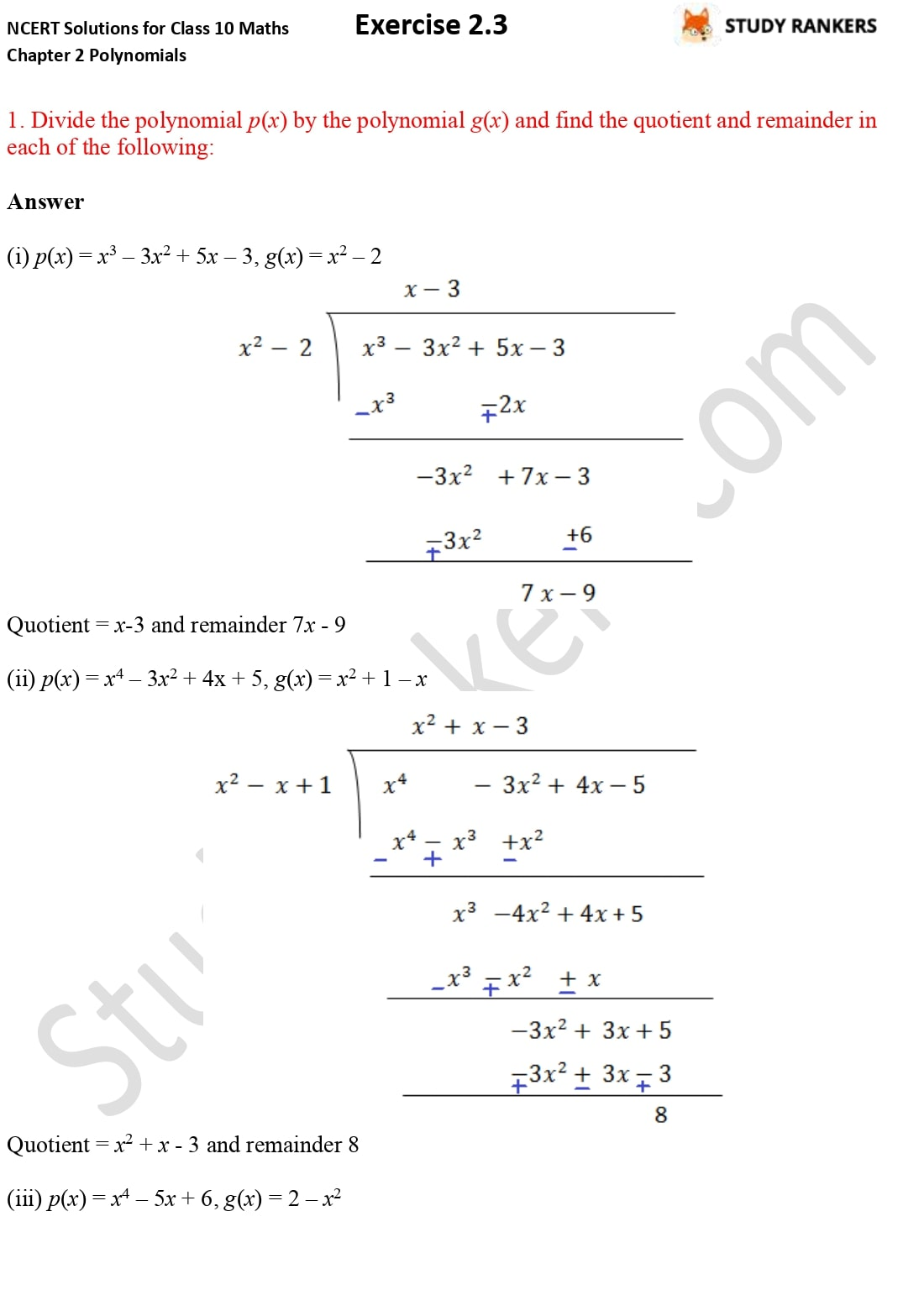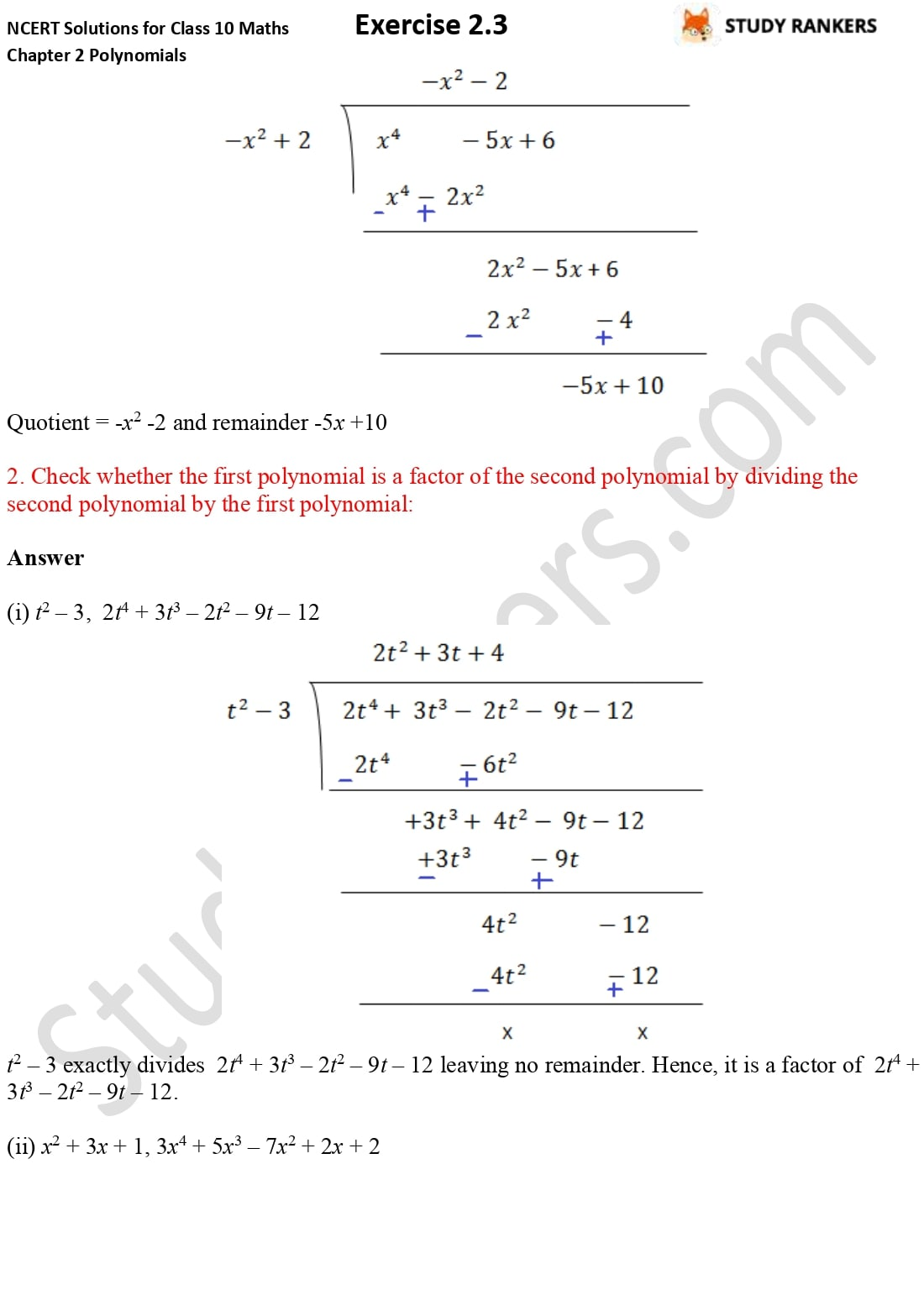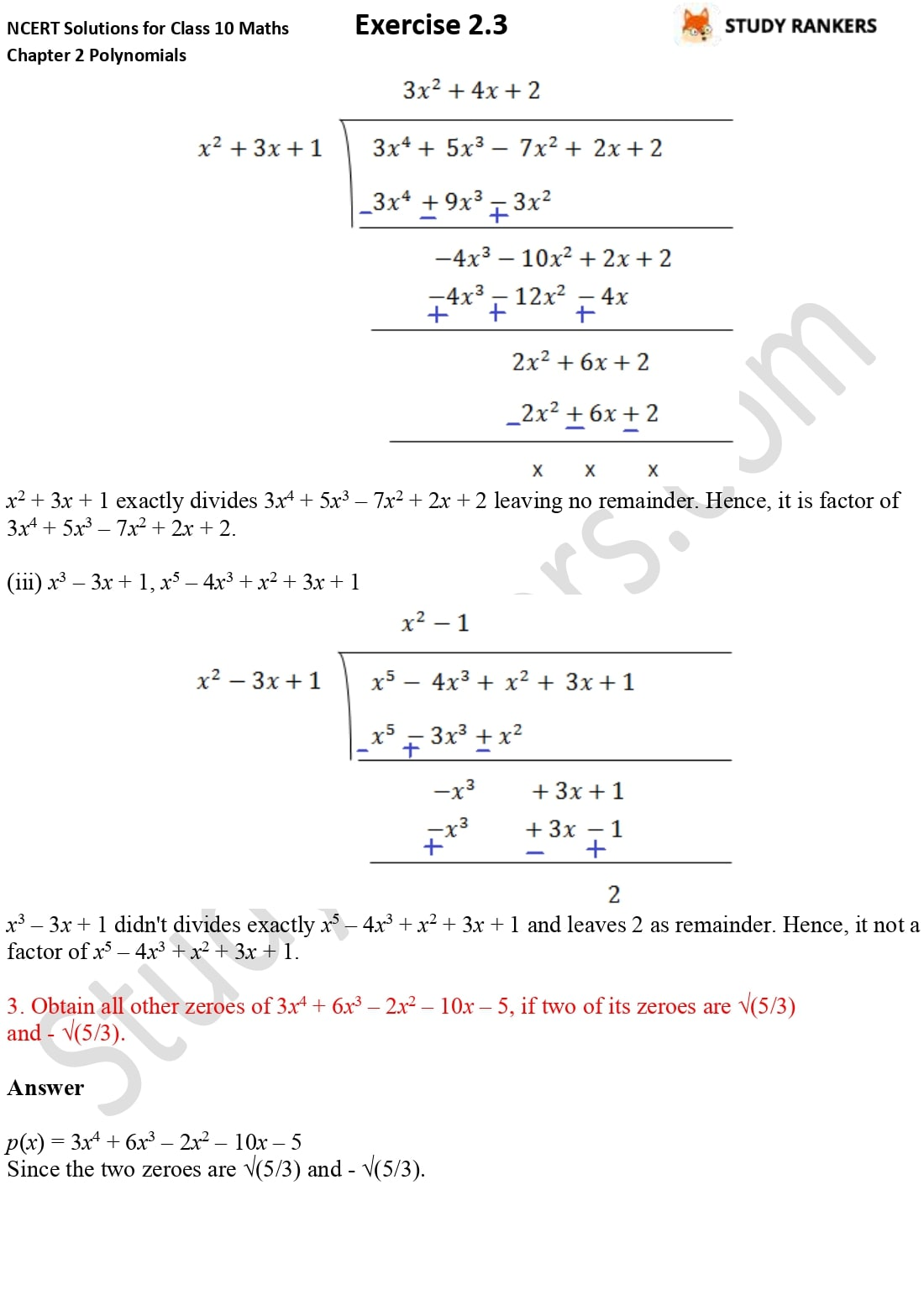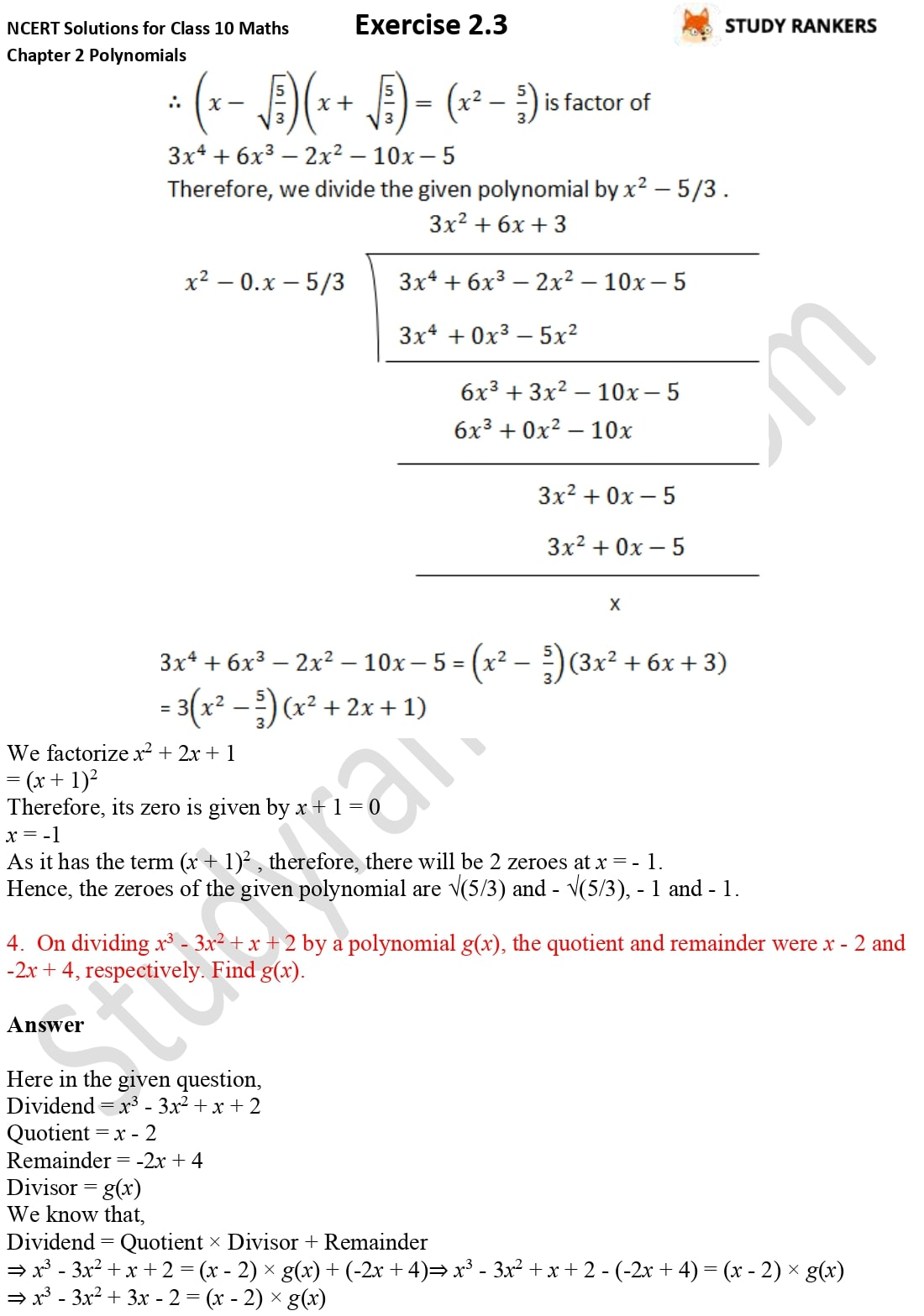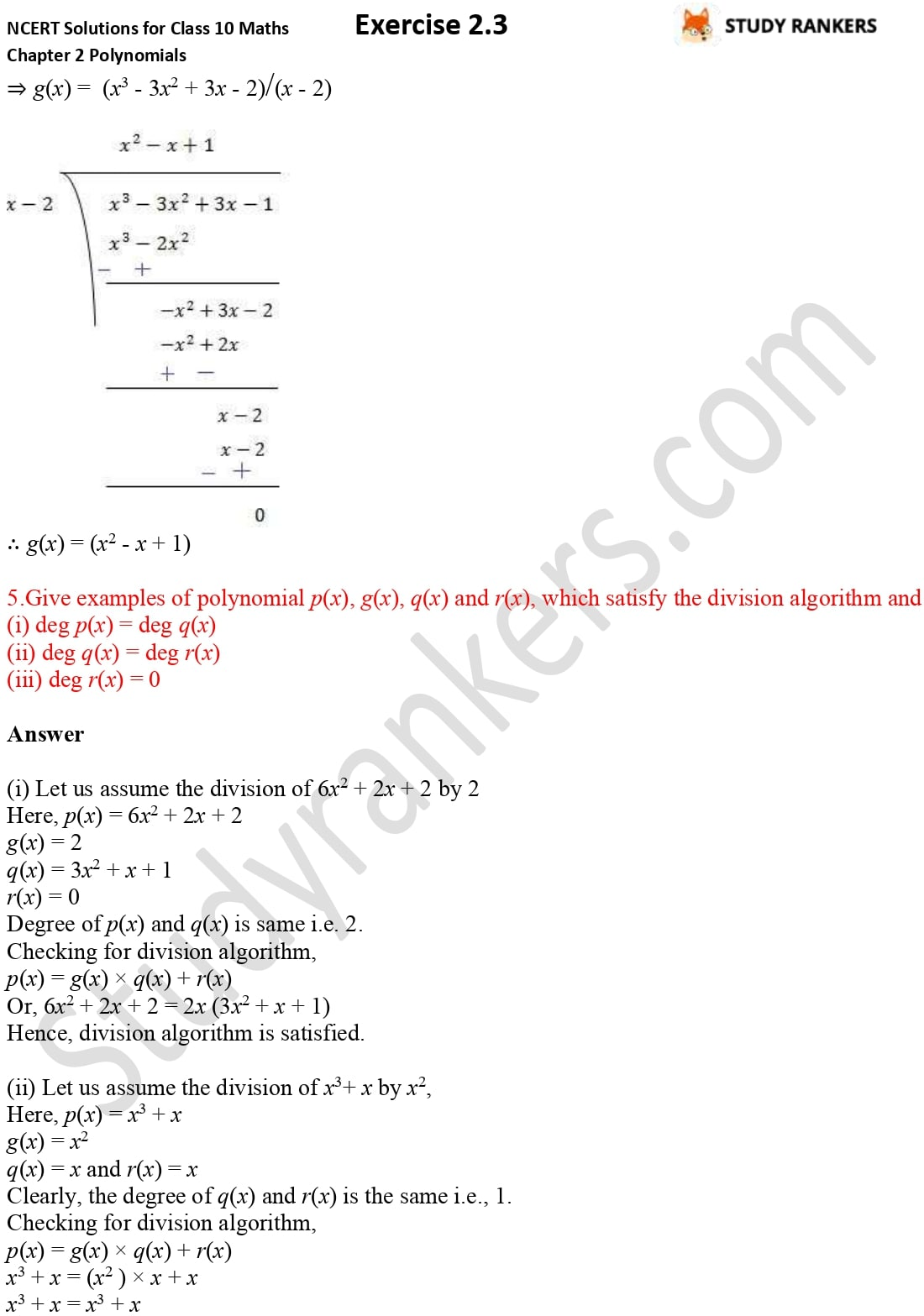>

## NCERT Solutions for Class 10 Maths Chapter 2 Polynomials Exercise 2.3

NCERT Solutions for Chapter 2 Polynomials Maths is provided here. You can improve your problem skills by practicing more and more questions. Before, going into advance books, you must try to solve NCERT Solutions for Class 10 Maths that will help you in understanding the concepts related the questions. These solutions are prepared by Studyrankers experts that are detailed and accurate.
There are total five questions given in the exercise in which you have to find the quotient and remainder by dividing the polynomial p(x) by the polynomial g(x) and obtain zeroes of polynomials.